Analysis WebNotes
arrow_back arrow_forward

# Class Contents

## Completeness

At various points in this course we have addressed the question: How can you tell that a sequence converges without knowing what it converges to? The best answer we have found so far was for monotonic sequences of real numbers:

A monotonic sequence converges if and only if it is bounded.

In the case of (sequentially) compact sets we also know a priori that any sequence has a convergent subsequence, although this does not directly address the convergence or otherwise of a particular sequence.

What sort of condition might tell us in more generality when a sequence converges? If we don't have a candidate for the limit available to work with, then we can't try to show that the terms of the sequence are getting closer and closer to a certain point. If, nevertheless, that is what they are doing, and we are looking for a test which will show this to us, then maybe we should think about the terms of the sequence getting closer and closer to each other. The following example shows that one has to be careful how one phrases this:

Example: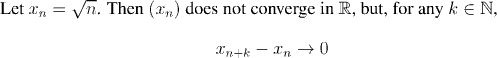The following definition is the right way to express the idea that the terms of a sequence are bunching together eventually.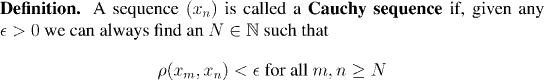Factoid:

Every convergent sequence is Cauchy.

Question: Prove this fact.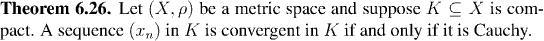Remark:

This is a very satisfactory characterization of when a sequence converges, in a compact set. However, although compact sets are very nice, one has to remember that the important sets of analysis are the real and complex number systems, which are not compact (although they have many compact subsets). It is useful that the same criterion (sometimes called the Cauchy Criterion) for when a sequence converges, can also be applied in the real and complex numbers.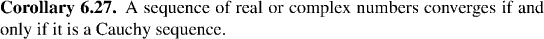Remark:
The proof relies on the fact that closed bounded sets in the real or complex numbers are compact. The key idea is to show that Cauchy sequences are always bounded, and from that we can show that a Cauchy sequence in R or C is always contained in a compact set. The result then follows from Theorem 6.27.

We have seen that Cauchy sequences always converge in compact metric spaces, and also in some which are not compact. Those metric spaces in which all Cauchy sequences converge are useful enough to deserve a special name: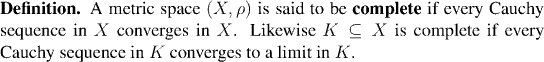Example: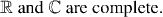Example: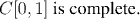Example: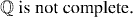In the next chapter, we are going to move away from abstract metric spaces, and work with functions in the real and complex number systems. Compactness and the properties of continuous functions on compact sets will still be importnat themes. Completeness will also play a key role. We shall be studying infinite series, and it is of crucial importance to be able to tell whether an infinite series converges, without knowing in advance what it converges to. Look out for completeness in the proof of the Comparison Test for series, which will be a key result in the coming chapter.